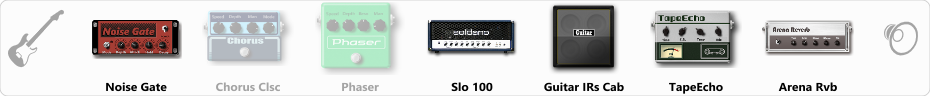# EVH

Discussion in 'ToneLib-GFX presets' started by Heavy Hand, Sep 25, 2019.

1. EVH

Preset name: Van Halen- L.Wo.A.N.

"Live Without a Net" tone

Effects chain:Effect: "Noise Gate" (Dynamics / Filter), active - "yes"
"Detect" = Gtr In
"Mode" = Manual
"Depth" = 50
"Threshold" = 68
"Attack" = 0
"Hold" = 5
"Decay" = 250

Effect: "Chorus Clsc" (Modulation / Sfx), active - "no"
"Speed" = 3.9
"Depth" = 28
"Center" = 5.2
"Mode" = Mono

Effect: "Phaser" (Modulation / Sfx), active - "no"
"Model" = MXR Phase 90
"Speed" = 3.4
"Depth" = 31
"Reso" = 18
"Freq" = 2.8

Effect: "Slo 100" (Amp simulators), active - "yes"
"Gain" = 58
"Bass" = 56
"Middle" = 66
"Treble" = 71
"Presence" = 41
"Master" = 44
"Output" = 50
"Level (dB)" = 5

Effect: "Guitar IRs Cab" (Cabinets), active - "yes"
"Model" = Marshall 1960A (4x12")
"Mic Position" = Middle
"Mic Distance" = Near
"Low Cut (Hz)" = 95
"Hi Cut (kHz)" = 12.5
"Mix" = 100
"Level (dB)" = 0

Effect: "TapeEcho" (Delay), active - "yes"
"Time" = 436
"Feedback" = 50
"Tone" = 27
"LoDamp" = 37
"Mix" = 47

Effect: "Arena Rvb" (Reverb), active - "yes"
"Time" = 7.5
"PreDelay" = 27
"LoDamp" = 28
"HiDamp" = 24
"Mix" = 47

Note: You will need to download and install the ToneLib-GFX software to use the preset.

#### Attached Files:

• ###### EVH.tlgfx
File size:
644 bytes
Views:
3,609
ARSZ, Manra, Ronvos and 3 others like this.